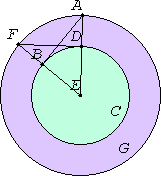# Proposition 17

From a given point to draw a straight line touching a given circle.

Let A be the given point, and BCD the given circle.It is required to draw from the point A a straight line touching the circle BCD.

Take the center E of the circle, and join AE. Describe the circle AFG with center E and radius EA. Draw DF from D at right angles to EA. Join EF and AB.

I say that AB has been drawn from the point A touching the circle BCD.

I.4

For, since E is the center of the circles BCD and AFG, EA equals EF, and ED equals EB. Therefore the two sides AE and EB equal the two sides FE and ED, and they contain a common angle, the angle at E, therefore the base DF equals the base AB, and the triangle DEF equals the triangle BEA, and the remaining angles to the remaining angles, therefore the angle EDF equals the angle EBA.

But the angle EDF is right, therefore the angle EBA is also right.

III.16,Cor

Now EB is a radius, and the straight line drawn at right angles to the diameter of a circle, from its end, touches the circle, therefore AB touches the circle BCD.

Therefore from the given point A the straight line AB has been drawn touching the circle BCD.

Q.E.F.

## Guide

The construction in this proposition is used in propositions III.34 and XII.2.